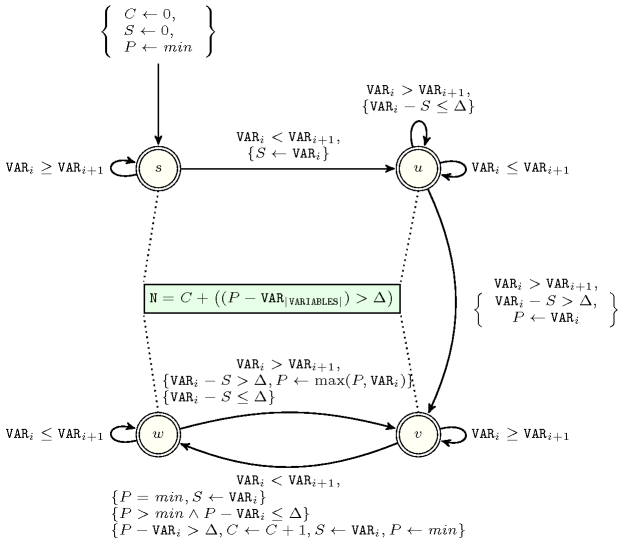## 5.51. big_peak

Origin

Derived from $\mathrm{𝚙𝚎𝚊𝚔}$.

Constraint

$\mathrm{𝚋𝚒𝚐}_\mathrm{𝚙𝚎𝚊𝚔}\left(𝙽,\mathrm{𝚅𝙰𝚁𝙸𝙰𝙱𝙻𝙴𝚂},\mathrm{𝚃𝙾𝙻𝙴𝚁𝙰𝙽𝙲𝙴}\right)$

Arguments
 $𝙽$ $\mathrm{𝚍𝚟𝚊𝚛}$ $\mathrm{𝚅𝙰𝚁𝙸𝙰𝙱𝙻𝙴𝚂}$ $\mathrm{𝚌𝚘𝚕𝚕𝚎𝚌𝚝𝚒𝚘𝚗}\left(\mathrm{𝚟𝚊𝚛}-\mathrm{𝚍𝚟𝚊𝚛}\right)$ $\mathrm{𝚃𝙾𝙻𝙴𝚁𝙰𝙽𝙲𝙴}$ $\mathrm{𝚒𝚗𝚝}$
Restrictions
 $𝙽\ge 0$ $2*𝙽\le \mathrm{𝚖𝚊𝚡}\left(|\mathrm{𝚅𝙰𝚁𝙸𝙰𝙱𝙻𝙴𝚂}|-1,0\right)$ $\mathrm{𝚛𝚎𝚚𝚞𝚒𝚛𝚎𝚍}$$\left(\mathrm{𝚅𝙰𝚁𝙸𝙰𝙱𝙻𝙴𝚂},\mathrm{𝚟𝚊𝚛}\right)$ $\mathrm{𝚃𝙾𝙻𝙴𝚁𝙰𝙽𝙲𝙴}\ge 0$
Purpose

A variable ${V}_{p}$ ($1) of the sequence of variables $\mathrm{𝚅𝙰𝚁𝙸𝙰𝙱𝙻𝙴𝚂}={V}_{1},\cdots ,{V}_{m}$ is a peak if and only if there exists an $i$ ($1) such that ${V}_{i-1}<{V}_{i}$ and ${V}_{i}={V}_{i+1}=\cdots ={V}_{p}$ and ${V}_{p}>{V}_{p+1}$. Similarly a variable ${V}_{v}$ ($1) is a valley if and only if there exists an $i$ $\left(1 such that ${V}_{i-1}>{V}_{i}$ and ${V}_{i}={V}_{i+1}=\cdots ={V}_{v}$ and ${V}_{v}<{V}_{v+1}$. A peak variable ${V}_{p}$ ($1) is a potential big peak wrt a non-negative integer $\mathrm{𝚃𝙾𝙻𝙴𝚁𝙰𝙽𝙲𝙴}$ if and only if:

1. ${V}_{p}$ is a peak,

2. $\exists i,j\in \left[1,m\right]|i, ${V}_{i}$ is a valley (or $i=1$ if there is no valley before position $p$), ${V}_{j}$ is a valley (or $i=m$ if there is no valley after position $p$), ${V}_{p}-{V}_{i}>\mathrm{𝚃𝙾𝙻𝙴𝚁𝙰𝙽𝙲𝙴}$, and ${V}_{p}-{V}_{j}>\mathrm{𝚃𝙾𝙻𝙴𝚁𝙰𝙽𝙲𝙴}$.

Let ${i}_{p}$ and ${j}_{p}$ be the largest $i$ and the smallest $j$ satisfying condition 2. Now a potential big peak ${V}_{p}$ ($1) is a big peak if and only if the interval $\left[i,j\right]$ does not contain any potential big peak that is strictly higher than ${V}_{p}$. The constraint $\mathrm{𝚋𝚒𝚐}_\mathrm{𝚙𝚎𝚊𝚔}$ holds if and only if $𝙽$ is the total number of big peaks of the sequence of variables $\mathrm{𝚅𝙰𝚁𝙸𝙰𝙱𝙻𝙴𝚂}$.

Example
 $\left(7,〈4,2,2,4,3,8,6,7,7,9,5,6,3,12,12,6,6,8,4,5,1〉,0\right)$ $\left(4,〈4,2,2,4,3,8,6,7,7,9,5,6,3,12,12,6,6,8,4,5,1〉,1\right)$

As shown part Part (A) of Figure 5.51.1, the first $\mathrm{𝚋𝚒𝚐}_\mathrm{𝚙𝚎𝚊𝚔}$ constraint holds since the sequence $42243867795631212668451$ contains seven big peaks wrt a tolerance of 0 (i.e., we consider standard peaks).

As shown part Part (B) of Figure 5.51.1, the second $\mathrm{𝚋𝚒𝚐}_\mathrm{𝚙𝚎𝚊𝚔}$ constraint holds since the same sequence $42243867795631212668451$ contains only four big peaks wrt a tolerance of 1.

##### Figure 5.51.1. Illustration of the Example slot: Part (A) a sequence of 21 variables ${V}_{1}$, ${V}_{2}$, ..., ${V}_{21}$ respectively fixed to values 4, 2, 2, 4, 3, 8, 6, 7, 7, 9, 5, 6, 3, 12, 12, 6, 6, 8, 4, 5, 1 and its corresponding 7 peaks ($\mathrm{𝚃𝙾𝙻𝙴𝚁𝙰𝙽𝙲𝙴}=0$ corresponds to standard peaks) with their respective heights ${h}_{1}^{0}=1$, ${h}_{2}^{0}=2$, ${h}_{3}^{0}=3$, ${h}_{4}^{0}=1$, ${h}_{5}^{0}=6$, ${h}_{6}^{0}=2$, ${h}_{7}^{0}=1$ (the left and right hand sides of each peak are coloured in light orange and light red) Part (B) the same sequence of variables and its 4 big peaks when $\mathrm{𝚃𝙾𝙻𝙴𝚁𝙰𝙽𝙲𝙴}=1$ with their respective heights ${h}_{1}^{1}=2$, ${h}_{2}^{1}=3$, ${h}_{3}^{1}=6$, ${h}_{4}^{1}=2$Typical
 $𝙽\ge 1$ $|\mathrm{𝚅𝙰𝚁𝙸𝙰𝙱𝙻𝙴𝚂}|>6$ $\mathrm{𝚛𝚊𝚗𝚐𝚎}$$\left(\mathrm{𝚅𝙰𝚁𝙸𝙰𝙱𝙻𝙴𝚂}.\mathrm{𝚟𝚊𝚛}\right)>1$ $\mathrm{𝚃𝙾𝙻𝙴𝚁𝙰𝙽𝙲𝙴}>1$
Symmetries
• Items of $\mathrm{𝚅𝙰𝚁𝙸𝙰𝙱𝙻𝙴𝚂}$ can be reversed.

• One and the same constant can be added to the $\mathrm{𝚟𝚊𝚛}$ attribute of all items of $\mathrm{𝚅𝙰𝚁𝙸𝙰𝙱𝙻𝙴𝚂}$.

Arg. properties
• Functional dependency: $𝙽$ determined by $\mathrm{𝚅𝙰𝚁𝙸𝙰𝙱𝙻𝙴𝚂}$ and $\mathrm{𝚃𝙾𝙻𝙴𝚁𝙰𝙽𝙲𝙴}$.

• Contractible wrt. $\mathrm{𝚅𝙰𝚁𝙸𝙰𝙱𝙻𝙴𝚂}$ when $𝙽=0$ and $\mathrm{𝚃𝙾𝙻𝙴𝚁𝙰𝙽𝙲𝙴}=0$.

Usage

Useful for constraining the number of big peaks of a sequence of domain variables, by ignoring too small valleys that artificially create small peaks wrt $\mathrm{𝚃𝙾𝙻𝙴𝚁𝙰𝙽𝙲𝙴}$.

specialisation: $\mathrm{𝚙𝚎𝚊𝚔}$ (the tolerance is set to 0 and removed).
Figure 5.51.2 depicts the automaton associated with the $\mathrm{𝚋𝚒𝚐}_\mathrm{𝚙𝚎𝚊𝚔}$ constraint. To each pair of consecutive variables $\left({\mathrm{𝚅𝙰𝚁}}_{i},{\mathrm{𝚅𝙰𝚁}}_{i+1}\right)$ of the collection $\mathrm{𝚅𝙰𝚁𝙸𝙰𝙱𝙻𝙴𝚂}$ corresponds a signature variable ${S}_{i}$. The following signature constraint links ${\mathrm{𝚅𝙰𝚁}}_{i}$, ${\mathrm{𝚅𝙰𝚁}}_{i+1}$ and ${S}_{i}$: $\left({\mathrm{𝚅𝙰𝚁}}_{i}<{\mathrm{𝚅𝙰𝚁}}_{i+1}⇔{S}_{i}=0\right)\wedge \left({\mathrm{𝚅𝙰𝚁}}_{i}={\mathrm{𝚅𝙰𝚁}}_{i+1}⇔{S}_{i}=1\right)\wedge \left({\mathrm{𝚅𝙰𝚁}}_{i}>{\mathrm{𝚅𝙰𝚁}}_{i+1}⇔{S}_{i}=2\right)$.
##### Figure 5.51.2. Automaton for the $\mathrm{𝚋𝚒𝚐}_\mathrm{𝚙𝚎𝚊𝚔}$ constraint where $C$, $S$, $P$, $\mathrm{𝑚𝑖𝑛}$ and $\Delta$ respectively stand for the number of big peaks already encountered, the altitude at the start of the current potential big peak, the altitude of the current potential big peak, the smallest value that can be assigned to a variable of $\mathrm{𝚅𝙰𝚁𝙸𝙰𝙱𝙻𝙴𝚂}$, the $\mathrm{𝚃𝙾𝙻𝙴𝚁𝙰𝙽𝙲𝙴}$ parameter